# 第 3 章 优化算法

## 3.2 牛顿优化算法

1. 选择初始猜测点 $$x_0$$，设置 $$n = 0$$
2. 按照以下迭代过程进行迭代：

$x_{n+1}=x_{n}-\frac{f^{\prime}\left(x_{n}\right)}{f^{\prime\prime}\left(x_{n}\right)}.$

1. 计算 $$|f^{\prime}(x_{n+1})|$$
1. 如果 $$|f^{\prime}(x_{n+1})| \leq \epsilon$$，停止迭代；
2. 否则，返回第 2 步。

• $$|x_{n} - x_{n-1}| < \epsilon$$.

• $$|f(x_{n}) - f(x_{n-1})| < \epsilon$$.

## 3.3 多元优化算法

$\nabla f(\mathbf x) = \frac{\partial f({\mathbf{x}})}{\partial {\mathbf{x}}} = \left( \begin{array}{c} \frac{\partial f(\mathbf x)}{\partial x_1}\\ \frac{\partial f(\mathbf x)}{\partial x_2}\\ \vdots\\ \frac{\partial f(\mathbf x)}{\partial x_d} \end{array} \right)$ 以及海塞矩阵

$\mathbf{H}(\mathbf x)= \frac{\partial^2 f({ \mathbf{x}})}{\partial {\mathbf{x}}\partial{\mathbf{x}}'}= \left(\begin{array}{cccc}\frac{\partial^{2} f(\mathbf x)}{\partial x_{1}^{2}} & \frac{\partial^{2} f(\mathbf x)}{\partial x_{1} \partial x_{2}} & \cdots & \frac{\partial^{2} f(\mathbf x)}{\partial x_{1} \partial x_{d}} \\ \frac{\partial^{2} f(\mathbf x)}{\partial x_{2} \partial x_{1}} & \frac{\partial^{2} f(\mathbf x)}{\partial x_{2}^{2}} & \cdots & \frac{\partial^{2} f(\mathbf x)}{\partial x_{2} \partial x_{d}} \\ \vdots & \vdots & \ddots & \vdots \\ \frac{\partial^{2} f(\mathbf x)}{\partial x_{d} \partial x_{1}} & \frac{\partial^{2} f(\mathbf x)}{\partial x_{d} \partial x_{2}} & \cdots & \frac{\partial^{2} f(\mathbf x)}{\partial x_{d}^{2}}\end{array}\right).$ 和一元优化问题类似，我们可以通过迭代求 $$f(\mathbf x)$$ 的极值。比如多元的牛顿优化算法的迭代过程可以写为：

$\mathbf{x_{n+1}}=\mathbf{x_n}-\left(\frac{\partial^2 f({ \mathbf{x}})}{\partial {\mathbf{x}}\partial{\mathbf{x}}'}\right)^{-1}\frac{\partial f({\mathbf{x}})}{\partial {\mathbf{x}}}.$ 停止条件可以为：

1. $$||\nabla f(\mathbf x_n)|| < \epsilon$$.

2. $$||\mathbf x_n - \mathbf x_{n-1}|| < \epsilon$$.

3. $$|f(\mathbf x_n) - f(\mathbf x_{n-1})|| < \epsilon$$.

newton <- function(f, x0, tol = 1e-9, max.iter = 100) {
x <- x0
cat(paste0('初始值：','x1 = ',x,', x2 = ',x,'\n'))
fx <- ftn(f, x)
iter <- 0
# xs用来保存每步迭代得到的x值
xs <- list()
xs[] <- x
# 继续迭代直到满足停止条件
while((max(abs(fx$fgrad)) > tol) & (iter < max.iter)){ x <- x - solve(fx$fhess, fx$fgrad) fx <- ftn(f, x) iter <- iter + 1 xs[[iter + 1]] <- x cat(paste0('迭代第', iter, '次：x1 = ', x, ', x2 = ', x, '\n')) } if (max(abs(fx$fgrad)) > tol){
cat('算法无法收敛 \n')
} else{
cat('算法收敛\n')
return(xs)
}
}

ftn <- function(f, x){
df <- deriv(body(f), c('x1', 'x2'), func = TRUE,
hessian = TRUE)
dfx <- df(x, x)
f <- dfx
fhess <- attr(dfx, 'hessian')[1,,]
}
f <- function(x1, x2) x1^2 - x1 * x2 + x2^2 + exp(x2)
#> 初始值：x1 = 5, x2 = 5
#> 迭代第1次：x1 = 1.97998841450638, x2 = 3.95997682901276
#> 迭代第2次：x1 = 1.4388441844607, x2 = 2.8776883689214
#> 迭代第3次：x1 = 0.865775331919303, x2 = 1.73155066383861
#> 迭代第4次：x1 = 0.289032888354303, x2 = 0.578065776708607
#> 迭代第5次：x1 = -0.114564289467867, x2 = -0.229128578935734
#> 迭代第6次：x1 = -0.212927882981344, x2 = -0.425855765962689
#> 迭代第7次：x1 = -0.21627797375019, x2 = -0.43255594750038
#> 迭代第8次：x1 = -0.216281377762501, x2 = -0.432562755525002
#> 算法收敛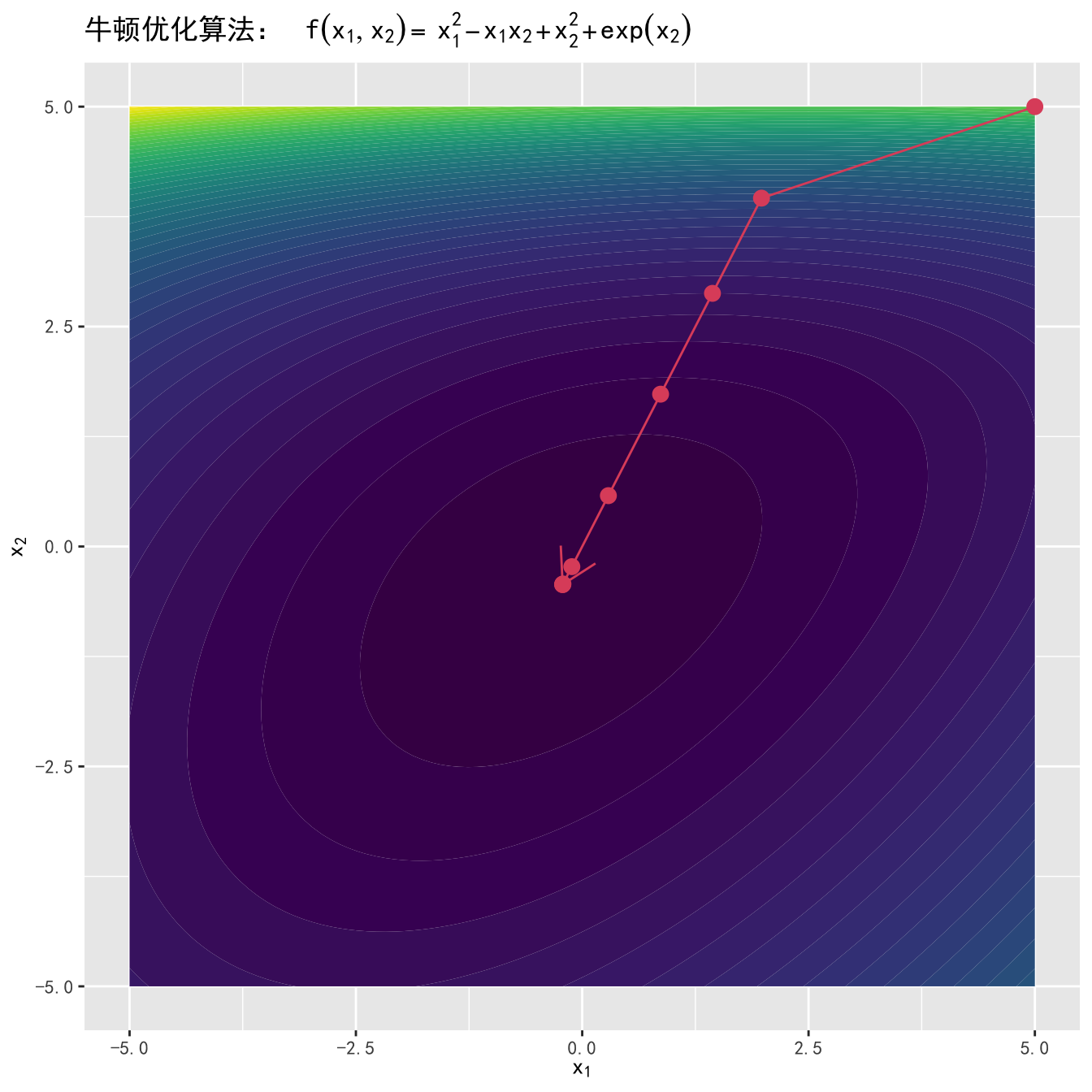Nelder Mead 算法的思路是计算目标函数 $$f(\cdot)$$$$n$$ 维单纯形顶点出的取值，其中 $$n$$$$f(\cdot)$$ 中的变量个数，所以 Nelder Mead 算法有时也被称为单纯形优化法。对于一个二维函数，单纯形即为三角形，三角形的每一个角是一个顶点。更一般的，$$n$$ 维单纯形有 $$n + 1$$ 个顶点。

3.1 展示了一个二维单纯形的例子。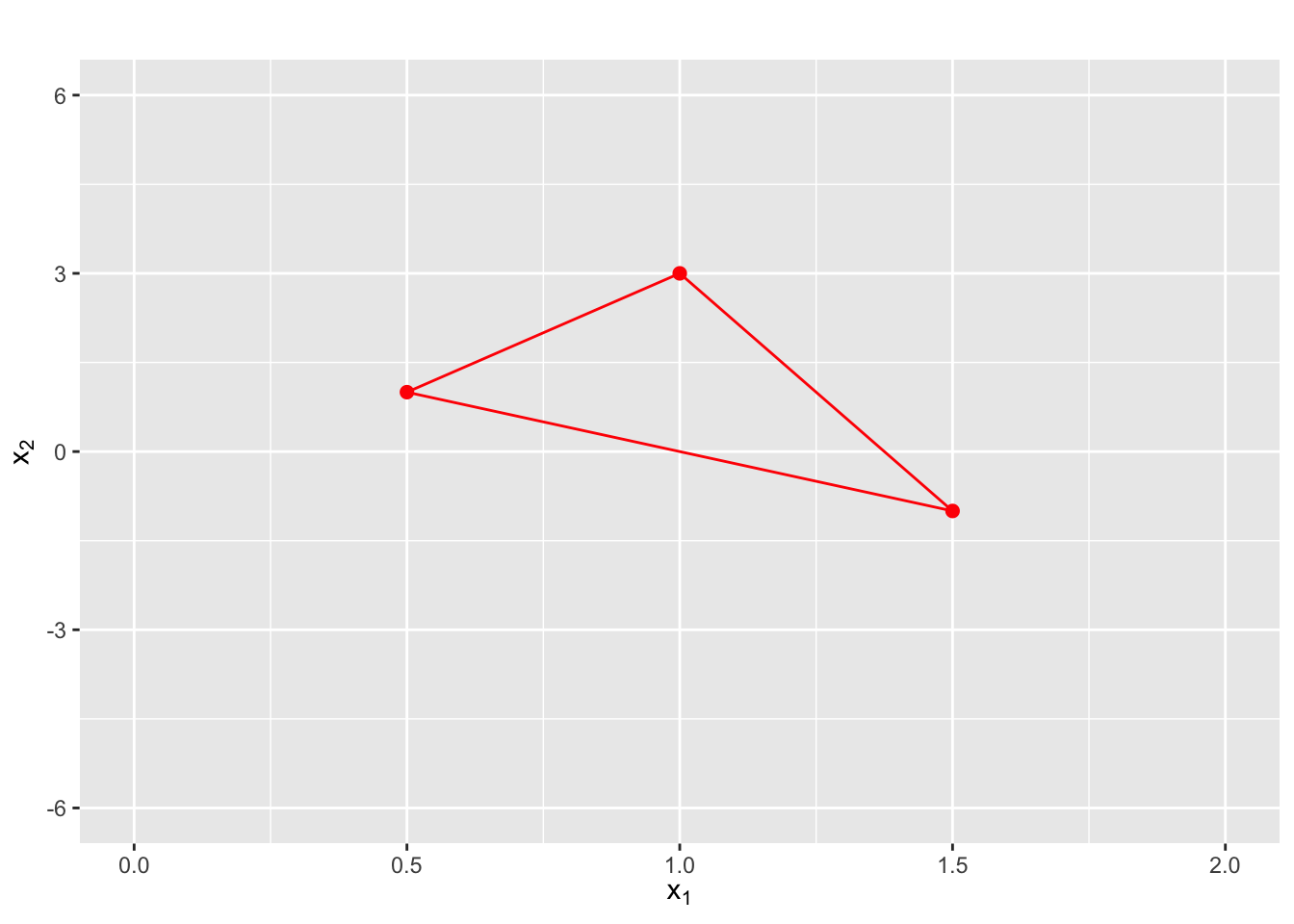#### 第一步：求函数值

$f({\mathbf x_1})\leq f({\mathbf x_2})\leq\ldots\leq f({\mathbf x_{n+1}}).$ 假设我们的目标是最小化 $$f(\cdot)$$，那么 $$f({\mathbf x_{n+1}})$$ 就是最差点。算法目标即使寻找更好的点来取代 $$f({\mathbf x_{n+1}})$$

#### 第二步：计算中心点

${\mathbf x_0}=\frac{1}{n}\sum_{j=1}^{n} {\mathbf x_j}.$ 对于一个二维单纯形，中点即为两点连线的中点。

#### 第三步：计算映射点

${\mathbf x_r}={\mathbf x_0}+\alpha({\mathbf x_0}-{\mathbf x_{n+1}}),$

1. $$f({\mathbf x_1})\leq f({\mathbf x_r})<f({\mathbf x_n})$$， 这种情况下 $${\mathbf x_r}$$ 既不是最佳点也不是最差点，将 $$\mathbf x_{n+1}$$ 替换为 $${\mathbf x_r}$$，形成新的单纯形，回到第一步。

2. $$f({\mathbf x_r})<f({\mathbf x_1})$$，即 $${\mathbf x_r}$$ 为最佳点，那么在这个方向上继续延伸，得到延伸点（Expansion Point）：

${\mathbf x_e}={\mathbf x_0}+\gamma({\mathbf x_r}-{\mathbf x_0})，$ 通常取 $$\gamma = 2$$。计算 $$f({\mathbf x_e})$$。 - 如果 $$f({\mathbf x_e}) < f({\mathbf x_r})$$，那么延伸点比映射点更优，用延伸点 $${\mathbf x_e}$$ 形成新的单纯形。 - 如果 $$f({\mathbf x_r})\leq f({\mathbf x_e})$$，用映射点 $${\mathbf x_r}$$ 形成新的单纯形。

1. $$f({\mathbf x_r})\geq f({\mathbf x_n})$$，即 $${\mathbf x_r}$$ 为最差点，这意味着在 $${\mathbf x_{n+1}}$$$${\mathbf x_{r}}$$ 之间可能存在谷点，这时寻找收缩点（contraction point）：

${\mathbf x_c}={\mathbf x_0}+\rho({\mathbf x_{n+1}}-{\mathbf x_0}),$ 通常取 $$\rho=0.5$$。 - 如果 $$f({\mathbf x_c}) < f({\mathbf x_{n+1}})$$，那么用收缩点 $${\mathbf x_c}$$ 形成新的单纯形。 - 如果 $$f({\mathbf x_c}) \geq f({\mathbf x_{n+1}})$$，说明收缩无效，需要一个更小的单纯形，这时将 $${\mathbf x_{1}}$$ 以外的点按以下方式压缩（shrink）：

${\mathbf x_i}={\mathbf x_1}+\sigma({\mathbf x_i}-{\mathbf x_1}),$ 通常取 $$\sigma = 0.5$$，得到新的单纯形后返回第一步。

3.2 展示了 Nelder Mead 算法优化函数 $$f(x_1, x_2) = x_1^2 + x_2^2 + x_1\sin(x_2) + x_2\sin(x_1)$$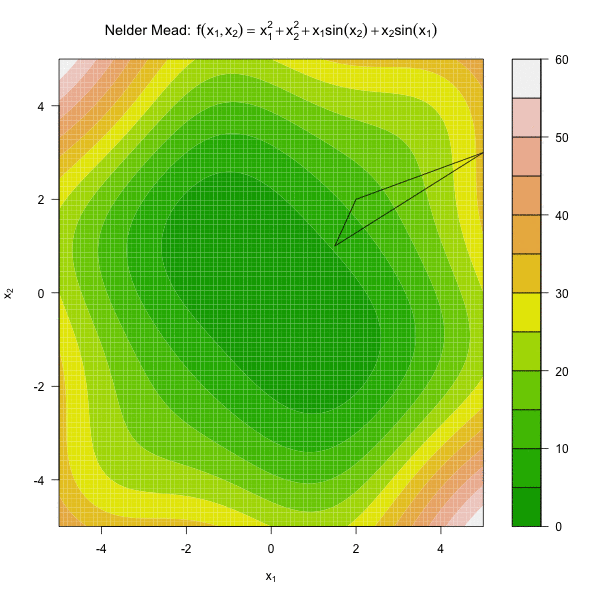#### 停止条件

$\frac{1}{2}|\mathrm{det}(\tilde{X})|,$ 其中

$\tilde{X}=\left({\mathbf x_1}-{\mathbf x_{n+1}},{\mathbf x_2-{\mathbf x_{n+1}}},\ldots, {\mathbf x_{n}-{\mathbf x_{n+1}}}\right).$

## 3.5 随机梯度下降算法

### 3.5.1 梯度下降算法

$\begin{equation} \mathbf{x}_{n+1}=\mathbf{x}_{n}-\alpha \nabla f\left(\mathbf{x}_{n}\right), \tag{3.1} \end{equation}$

$\begin{equation} g(\alpha) = f(\mathbf{x}_n - \alpha \nabla f(\mathbf{x}_n), \tag{3.2} \end{equation}$ 如果我们的初始猜测为 $$\mathbf{x}_0$$，那么根据公式 (3.1)，我们可以得到 $$f(\mathbf{x}_0) \geq f(\mathbf{x}_1) \geq f(\mathbf{x}_2) \geq \cdots$$，如果算法收敛，序列 $$\{\mathbf{x}_n\}$$ 就会收敛到 $$f(\mathbf{x})$$ 的局部极小值。所以我们可以以 $$f(\mathbf{x}_{n+1})- f(\mathbf{x}_n) \leq \epsilon$$ 为梯度下降算法收敛的停止条件，其中 $$\epsilon$$ 为容忍值。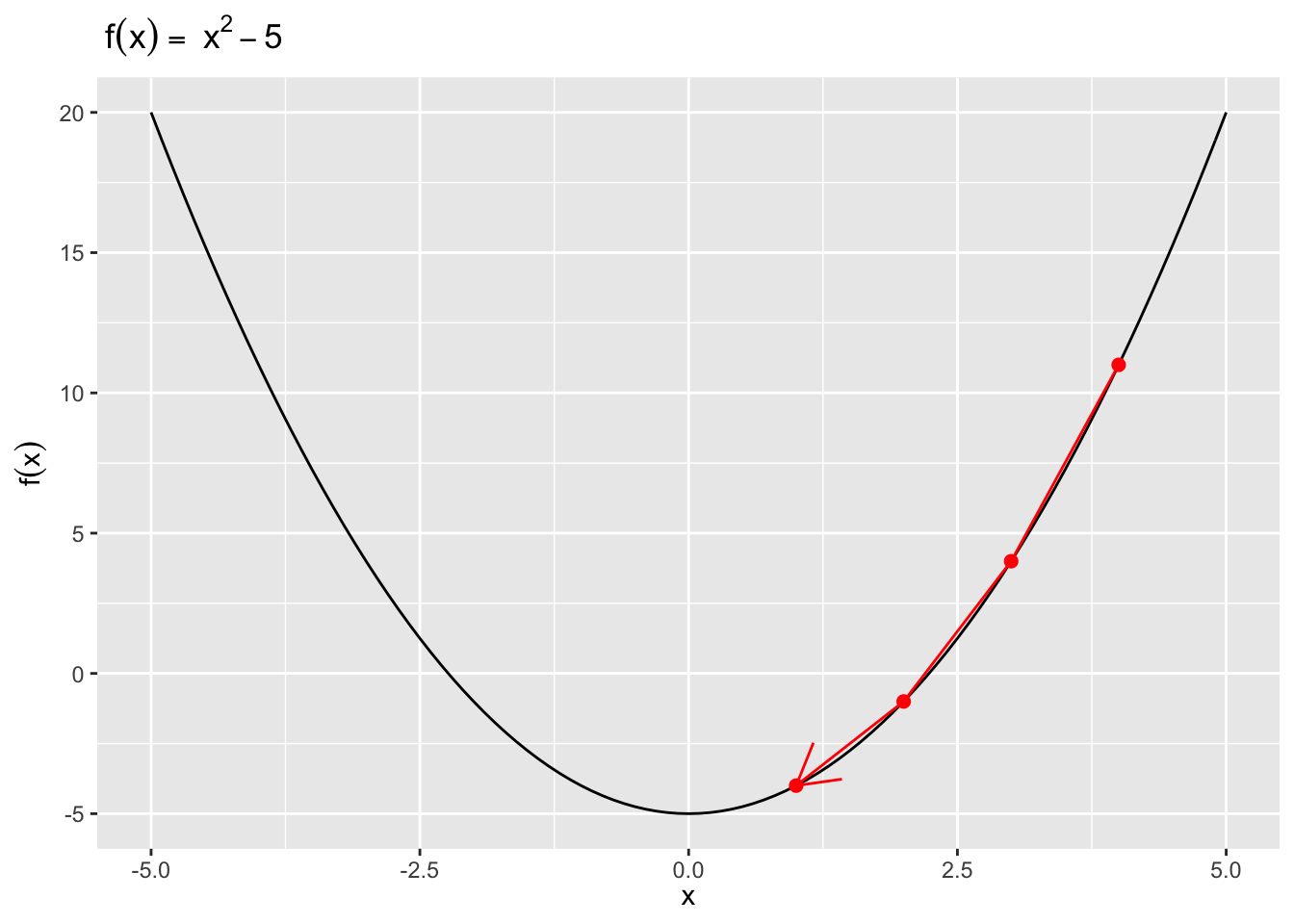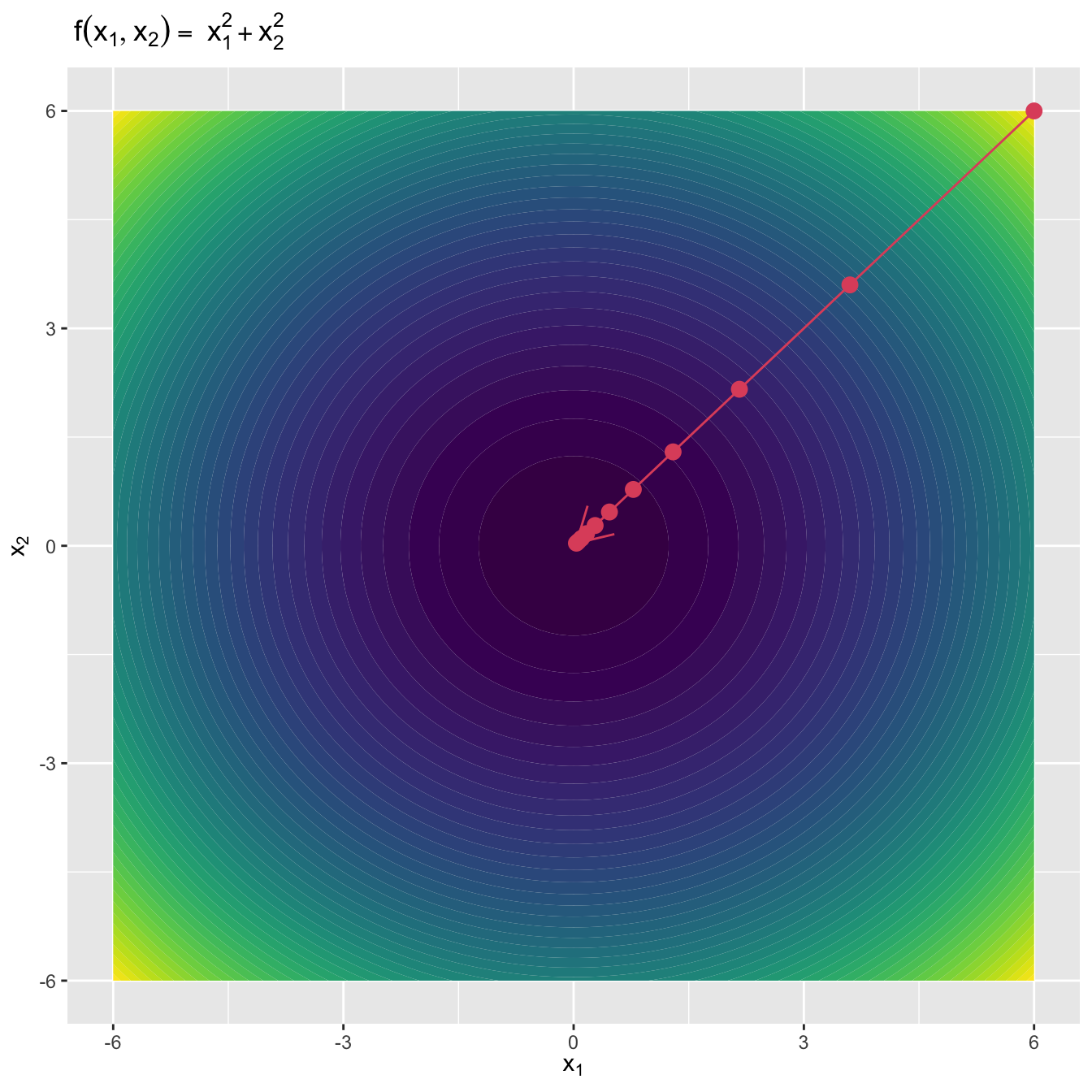$J(\beta) = \frac{1}{2n}(X\beta - y)^T(X\beta - y).$ 对应的梯度向量为

$\nabla J(\beta) = \frac{1}{n}X^T(X\beta-y).$

gd.lm <- function(X, y, beta.init, alpha, tol = 1e-5,
max.iter = 100){
beta.old <- beta.init
J <- betas <- list()
if (alpha == 'auto'){
alpha <-
optim(0.1, function(alpha) {
lm.cost(
X, y,
beta.old - alpha * lm.cost.grad(X, y, beta.old))
}, method = 'L-BFGS-B', lower = 0, upper = 1)
if (alpha$convergence == 0) { alpha <- alpha$par
} else{
alpha <- 0.1
}
}
betas[] <- beta.old
J[] <- lm.cost(X, y, beta.old)
beta.new <- beta.old -
betas[] <- beta.new
J[] <- lm.cost(X, y, beta.new)
iter <- 0
while ((abs(lm.cost(X, y, beta.new) -
lm.cost(X, y, beta.old))  > tol) &
(iter < max.iter)) {
beta.old <- beta.new
if (alpha == 'auto'){
alpha <-
optim(0.1, function(alpha) {
lm.cost(X, y, beta.old -
method = 'L-BFGS-B', lower = 0, upper = 1)
if (alpha$convergence == 0) { alpha <- alpha$par
} else{
alpha <- 0.1
}
}
beta.new <- beta.old -
iter <- iter + 1
betas[[iter + 2]] <- beta.new
J[[iter + 2]] <- lm.cost(X, y, beta.new)
}
if (abs(lm.cost(X, y, beta.new) -
lm.cost(X, y, beta.old)) > tol){
cat('算法无法收敛 \n')
} else{
cat('算法收敛\n')
cat('共迭代',iter + 1,'次','\n')
cat('系数估计为：', beta.new, '\n')
return(list(coef = betas,
cost = J,
niter = iter + 1))
}
}
## Generate some data
beta0 <- 1
beta1 <- 3
sigma <- 1
n <- 10000
x <- rnorm(n, 0, 1)
y <- beta0 + x * beta1 + rnorm(n, mean = 0, sd = sigma)
X <- cbind(1, x)

## Make the cost function
lm.cost <- function(X, y, beta){
n <- length(y)
loss <- sum((X%*%beta -  y)^2)/(2*n)
return(loss)
}
n <- length(y)
(1/n)*(t(X)%*%(X%*%beta - y))
}
## Use optimized alpha
gd.auto <- gd.lm(X, y, beta.init = c(-4,-5), alpha = 'auto',
tol = 1e-5, max.iter = 10000)
#> 算法收敛
#> 共迭代 3 次
#> 系数估计为： 0.9939 3

## alpha = 0.1
gd1 <- gd.lm(X, y, beta.init = c(-4,-5), alpha = 0.1,
tol = 1e-5, max.iter = 10000)
#> 算法收敛
#> 共迭代 67 次
#> 系数估计为： 0.9896 2.993

## alpha = 0.01
gd2 <- gd.lm(X, y, beta.init = c(-4,-5), alpha = 0.01,
tol = 1e-5, max.iter = 10000)
#> 算法收敛
#> 共迭代 570 次
#> 系数估计为： 0.9778 2.973
niter <- data.frame(alpha = c('auto', 0.1, 0.01),
niter = c(gd.auto$niter, gd1$niter,
gd2$niter)) knitr::kable( niter, caption = '不同$\\alpha$对应的梯度下降算法的迭代次数', booktabs = TRUE, col.names = c("$\\alpha", '迭代次数'), ) 表3.1: 不同 $$\alpha$$ 对应的梯度下降算法的迭代次数 $$\alpha$$ 迭代次数 auto 3 0.1 67 0.01 570 从表3.1中我们可以发现在每次迭代过程中对 $$\alpha$$ 进行优化可以显著提高计算效率。如图3.6，如果 $$\alpha$$ 较小，迭代的次数就会增加，因此收敛过程比较缓慢。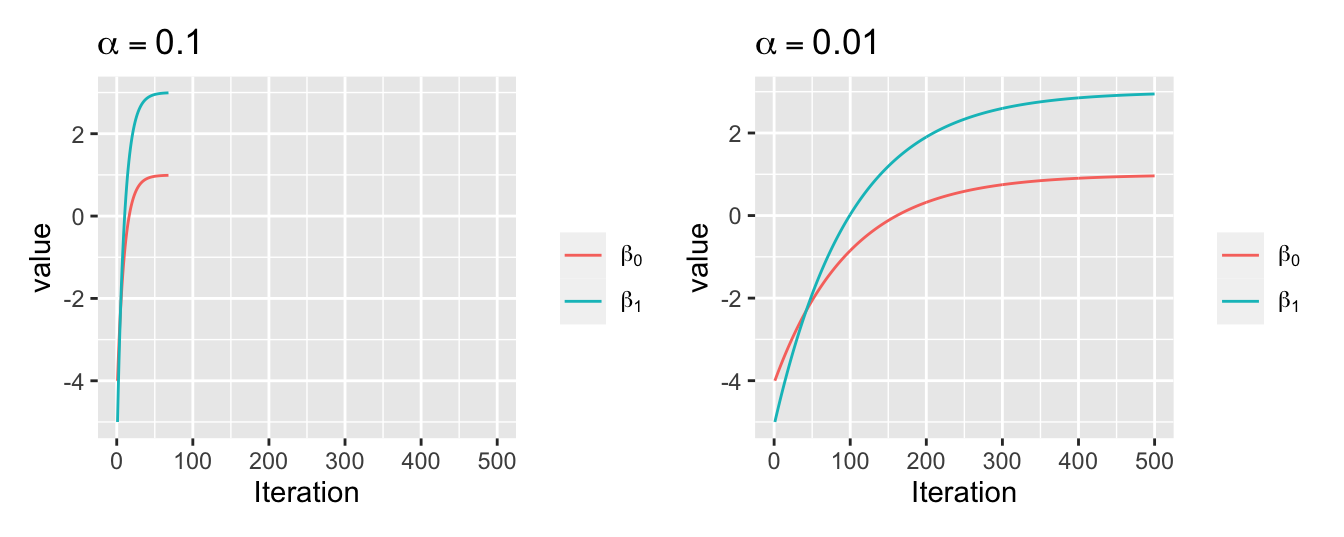图3.6: 不同 $$\alpha$$ 取值下梯度下降算法的收敛情况 ### 3.5.2 随机梯度下降算法 梯度下降算法通常收敛速度较慢，因为在每次迭代中对每个样本值都需要计算梯度，在样本量较大时计算量会随之增大。随机梯度下降算法的思想是在算法的每次迭代更新时只考虑一个样本。以线性回归模型为例，就是将梯度下降算法中的 $\nabla J(\beta) = \frac{1}{n}X^T(X\beta-y).$ 替换为 ： $\nabla J(\beta)_i = X_i^T(X_i\beta-y_i),$ 完整的迭代过程如下。 随机梯度下降算法 • 将训练数据随机排序，然后重复第2步直至收敛。 • 抽取一个随机样本 $$i\in\{1, 2, \cdots, n\}$$，根据下式对 $$\beta$$ 进行迭代。 $\beta:= \beta - \alpha \nabla J(\beta)_i.$ 随机梯度下降在R中的实现如下。 sgd.lm <- function(X, y, beta.init, alpha = 0.5, n.samples = 1, tol = 1e-5, max.iter = 100){ n <- length(y) beta.old <- beta.init J <- betas <- list() sto.sample <- sample(1:n, n.samples, replace = TRUE) betas[] <- beta.old J[] <- lm.cost(X, y, beta.old) beta.new <- beta.old - alpha * sgd.lm.cost.grad(X[sto.sample, ], y[sto.sample], beta.old) betas[] <- beta.new J[] <- lm.cost(X, y, beta.new) iter <- 0 n.best <- 0 while ((abs(lm.cost(X, y, beta.new) - lm.cost(X, y, beta.old)) > tol) & (iter + 2 < max.iter)){ beta.old <- beta.new sto.sample <- sample(1:n, n.samples, replace = TRUE) beta.new <- beta.old - alpha * sgd.lm.cost.grad(X[sto.sample, ], y[sto.sample], beta.old) iter <- iter + 1 betas[[iter + 2]] <- beta.new J[[iter + 2]] <- lm.cost(X, y, beta.new) } if (abs(lm.cost(X, y, beta.new) - lm.cost(X, y, beta.old)) > tol){ cat('算法无法收敛。 \n') } else{ cat('算法收敛\n') cat('共迭代',iter + 1,'次','\n') cat('系数估计为：', beta.new, '\n') return(list(coef = betas, cost = J, niter = iter + 1)) } } ## Make the cost function sgd.lm.cost <- function(X, y, beta){ n <- length(y) if (!is.matrix(X)){ X <- matrix(X, nrow = 1) } loss <- sum((X%*%beta - y)^2)/(2*n) return(loss) } ## Calculate the gradient sgd.lm.cost.grad <- function(X, y, beta){ n <- length(y) if (!is.matrix(X)){ X <- matrix(X, nrow = 1) } t(X)%*%(X%*%beta - y)/n } 现在我们在章节3.5.1中模拟的线性回归数据中实现SGD算法。 #> 算法收敛 #> 共迭代 253 次 #> 系数估计为： 1.157 2.95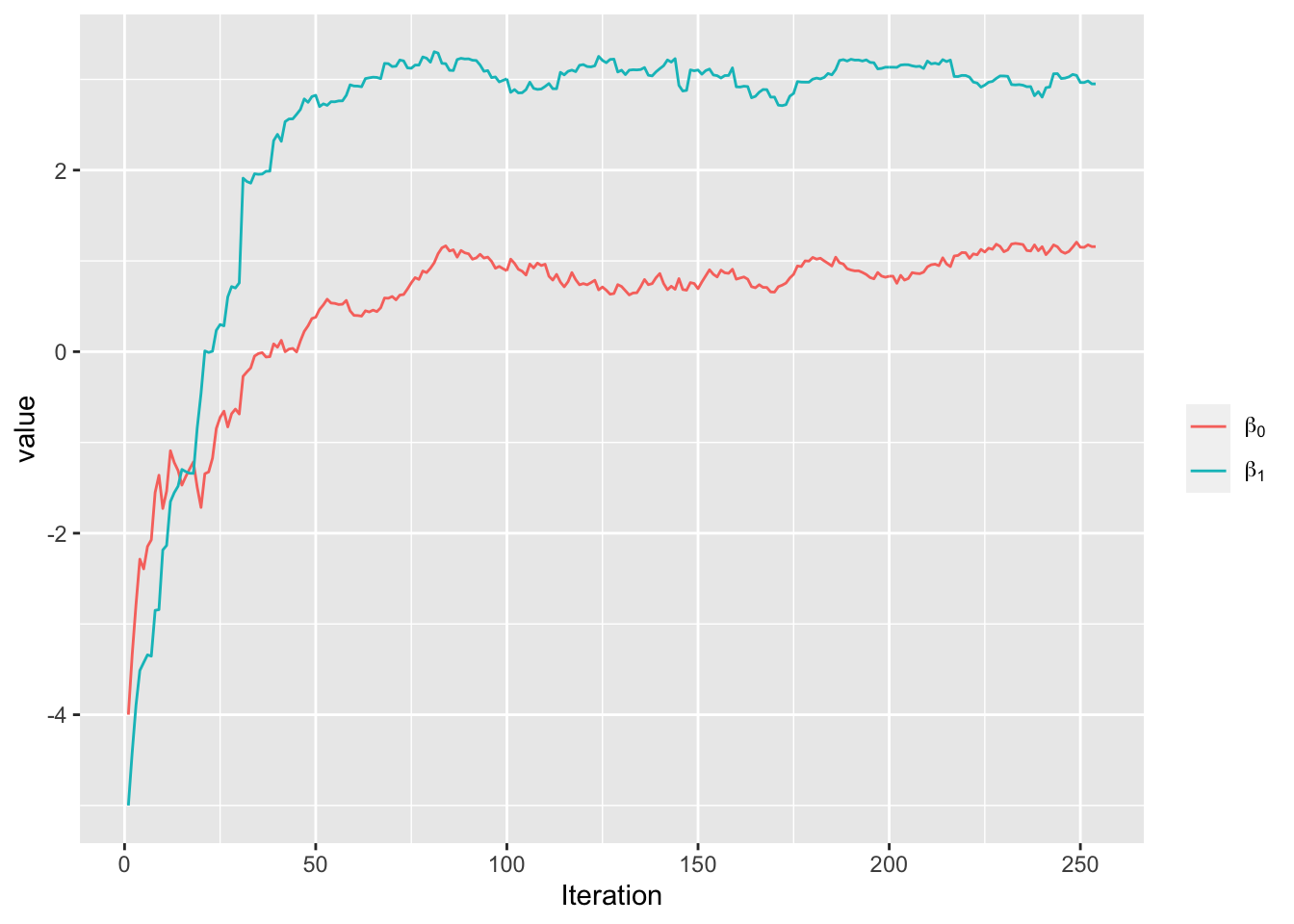图3.7: 随机梯度下降算法的收敛情况 ## 3.6 优化算法比较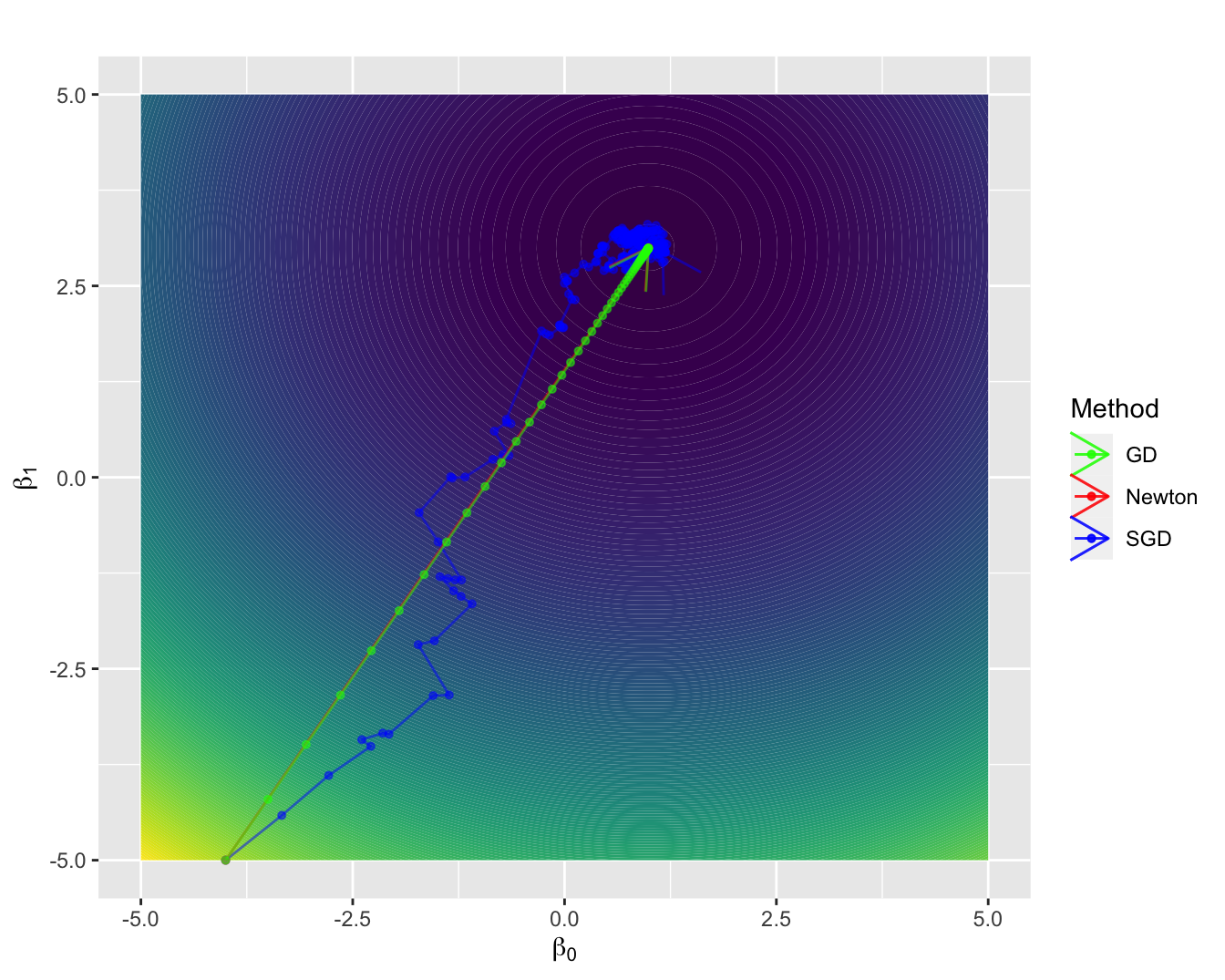图3.8: 梯度下降算法、随机梯度下降算法和牛顿算法的收敛情况比较 基于章节3.5.1中模拟的线性回归数据，我们来比较梯度下降算法、随机梯度下降算法和牛顿算法。从图3.8中我们可以发现： 1. 牛顿迭代的收敛速度很快，前提条件是海塞矩阵存在，但在复杂的机器学习方法中海塞矩阵的解析表达式通常很难求得或者无法求得。 2. 如果训练样本的个数非常庞大，牛顿方法或者梯度下降方法可能会花费很长的时间才可以收敛，这时随机梯度下降是一个更好的选择。 3. 随机梯度下降的收敛路径带有较多噪音，这是因为SGD的每次迭代只用了一个随机样本，为了平衡SGD带来的不确定性和GD的计算复杂度，我们可以在每步迭代时抽取少量样本，通常可以达到更加稳健的收敛，这也就是 mini-batch GD。 4. 对于 $$\alpha$$ 的选择，可以参考 Bottou (2012)Zeiler (2012) ## 3.7 案例：心脏病预测 #### Logistic回归 Logistic 回归是应用最为广泛的分类算法之一，它通常用于解决二分类问题（0或1），例如垃圾邮件识别、疾病诊断等应用。Logistic 回归模型假定观测 $$y$$ 服从概率为 $$p$$的伯努利分布，该算法的模型设定如下： $\hat p=g(\beta^Tx) = g(\beta_0+\beta_1x_1+\dots,\beta_kx_k)$ 其中 $$g(x)=\frac{1}{1+e^{-x}}$$ 为链接函数，它的主要目的是将 $$\beta^Tx$$$$(-\infty,+\infty)$$ 映射到 $$(0,1)$$，当 $$\hat p>0.5$$ 时，$$\hat y=1$$，当 $$\hat p<0.5$$ 时，$$\hat y=0$$ Logistic 回归模型常采用极大似然估计法进行估计，可以写出它的对数似然函数如下： \begin{align*} L &= \mathrm{log}\left[\prod_{i=1}^np_i^{y_i}(1-p_i)^{1-y_i}\right]\\\ &=\sum_{i=1}^n\left[y_i\mathrm{log}p_i+(1-y_i)\mathrm{log}(1-p_i)\right]\\\ &=\sum_{i=1}^n\left[y_i\beta^Tx_i-\mathrm{log}(1+e^{\beta^Tx_i})\right]. \end{align*} 接下来我们使用本章之前讲过的随机梯度下降算法，结合实际的心脏病发病数据，进行基于 Logistic 回归模型的拟合和预测。 #### Logistic 回归随机梯度下降算法的 R 实现 sgd.logisticReg <- function(X, y, beta.init, alpha = 0.5, n.samples = 1, tol = 1e-5, max.iter = 100){ n <- length(y) beta.old <- beta.init J <- betas <- list() sto.sample <- sample(1:n, n.samples, replace = TRUE) # sto.sample <- 1:n betas[] <- beta.old J[] <- sgd.logisticReg.cost(X, y, beta.old) beta.new <- beta.old - alpha * sgd.logisticReg.cost.grad(X[sto.sample, ], y[sto.sample], beta.old) betas[] <- beta.new J[] <- sgd.logisticReg.cost(X, y, beta.new) iter <- 0 n.best <- 0 while ((abs(sgd.logisticReg.cost(X, y, beta.new) - sgd.logisticReg.cost(X, y, beta.old)) > tol) & (iter + 2 < max.iter)){ beta.old <- beta.new sto.sample <- sample(1:n, n.samples, replace = TRUE) beta.new <- beta.old - alpha * sgd.logisticReg.cost.grad(X[sto.sample, ], y[sto.sample], beta.old) iter <- iter + 1 betas[[iter + 2]] <- beta.new J[[iter + 2]] <- sgd.logisticReg.cost(X, y, beta.new) } if (abs(sgd.logisticReg.cost(X, y, beta.new) - sgd.logisticReg.cost(X, y, beta.old)) > tol){ cat('算法无法收敛。 \n') } else{ cat('算法收敛\n') cat('共迭代',iter + 1,'次','\n') # cat('系数估计为：', beta.new, '\n') return(list(coef = betas, cost = J, niter = iter + 1)) } } ## Make the cost function sgd.logisticReg.cost <- function(X, y, beta){ n <- length(y) if (!is.matrix(X)){ X <- matrix(X, nrow = 1) } p <- 1/(1+exp(-X %*% beta)) loss <- -sum(y*log(p)+(1-y)*log(1-p))/n return(loss) } ## Calculate the gradient sgd.logisticReg.cost.grad <- function(X, y, beta){ n <- length(y) if (!is.matrix(X)){ X <- matrix(X, nrow = 1) } t(X)%*%(1/(1+exp(-X%*%beta)) - y)/n } #### 心脏病预测 本案例采用的数据来源于Kaggle平台，主要目标是根据吸烟数、年龄、性别、相关疾病、身体健康指数等预测病人是否会有心脏病。 hd = read.csv("data/framingham_heart_disease.csv") #### 拟合Logistic回归 为了加快随机梯度下降速度，对部分特征进行标准化，数据共有3656条，用其中的3000条进行训练，剩余的用作测试。 mean_norm <- function(x){ (x-mean(x))/sd(x) } X <- cbind(1, as.matrix(hd[,-14])) y <- as.matrix(hd[,14]) X[,3] <- mean_norm(X[,3]) X[,4] <- log(X[,4]+1) X[,9:14] <- apply(X[,9:14], 2, mean_norm) X_train <- X[1:3000,] y_train <- y[1:3000,] X_test <- X[3001:3656,] y_test <- y[3001:3656,] beta.init <- runif(14, -1, 0) res <- sgd.logisticReg(X_train, y_train, beta.init, alpha = 0.01, n.samples = 1, max.iter = 1000, tol = 1e-6) #> 算法收敛 #> 共迭代 540 次 plot(1:length(rescost), res$cost, type = "l", xlab = "Iterations", ylab = "Loss")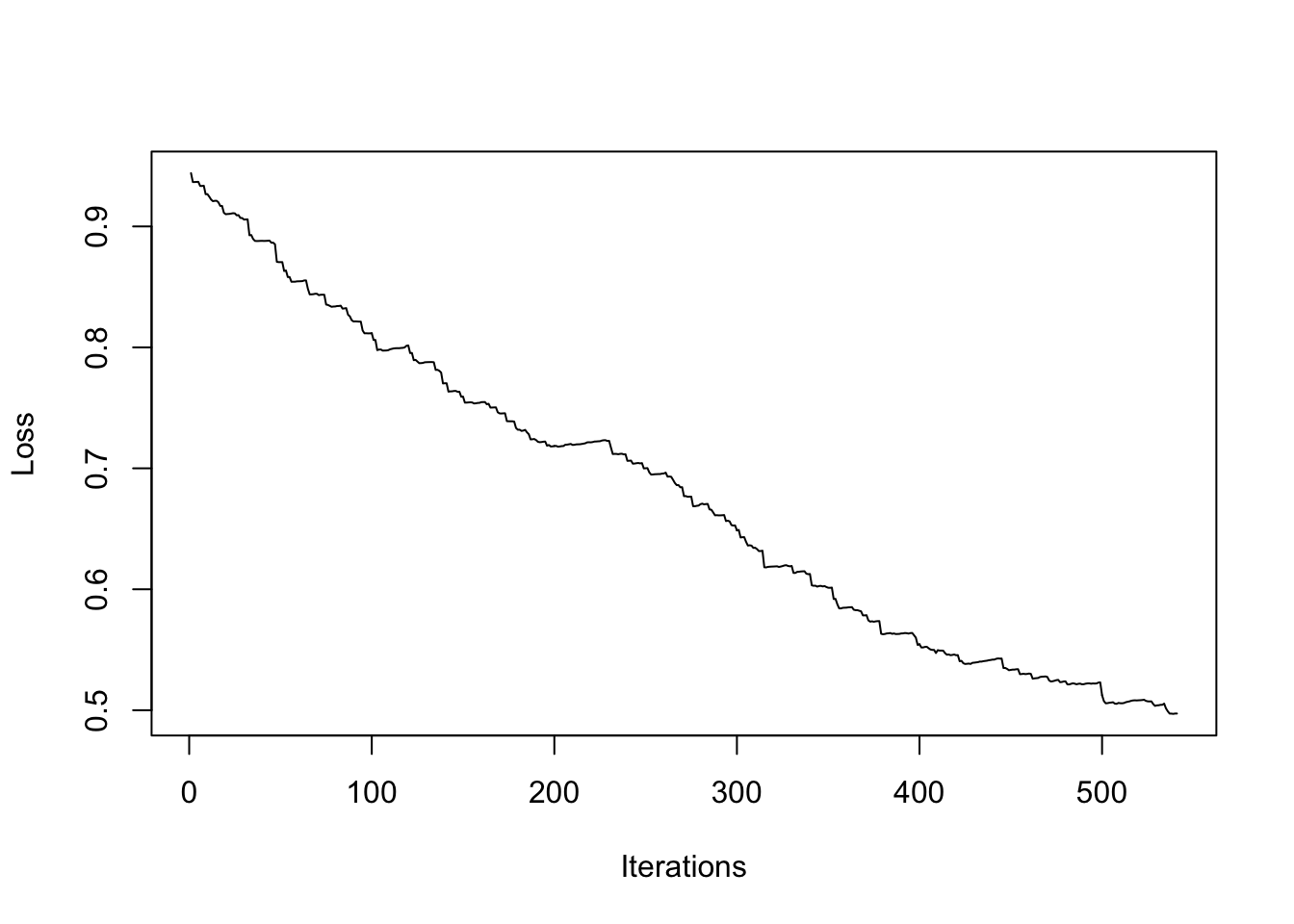#### 预测 p <- 1/(1+exp(-X_test%*%res$coef[[length(res\$coef)]]))
y_predicted <- ifelse(p>=0.5, 1, 0)
print(paste0("accuracy:", sum(y_predicted==y_test)/656))
#>  "accuracy:0.836890243902439"

## 3.8 拓展阅读

• Bottou (2012) 是实战中随机梯度下降算法通常用到的一些技巧。
• Zeiler (2012) 是关于如何适应性的选择梯度下降算法和随机梯度下降算法中的 $$\alpha$$

### 参考文献

Bottou, Léon. 2012. “Stochastic Gradient Descent Tricks.” In Neural Networks: Tricks of the Trade, 421–36. Springer.